Mathematics

1.2k views

 1 Introduction 2 What is a Quadratic Function? 3 Quadratic Parent Function 4 Inverse of a Quadratic Function 5 What are the Properties of Quadratic Function? 6 Summary 7 FAQs

30 October  2020

## Introduction

What is the inverse of a quadratic function?

When you're working during a physics laboratory for a category, you'll often end up making graphs of a few variables. You change one of the variables yourself and track the corresponding change in the other.

For example, you could be tasked with placing a sealed container filled with gas in a pot of water and measuring the change in pressure of the gas as the water is heated.

Here, the temperature of the water is that the variable you're changing, and therefore the pressure is that of the second variable you're tracking. When you make a graph of pressure vs. temperature you are exploring the relationship between these two variables.There are four different common relationships between variables you're sure to run into: they're linear, direct, quadratic, and inverse relationships. Here, we'll go over both quadratic and inverse relationships, and a couple examples .

In the amazing world of algebra, there is a fascinating topic called Quadratic polynomial, Quadratic functions etc. Fun explodes with the solving of equations, making graphs along with understanding the real life and practical use of this function.

## What is a Quadratic Function?

A quadratic function is one of the form f(x) = ax2 + bx + c, where a, b, and c are numbers with a not equal to zero and constants.

The shape of the graph of a quadratic function is a curve known as a parabola. Parabolas might open upward or downward, but they all have the same basic "U" shape.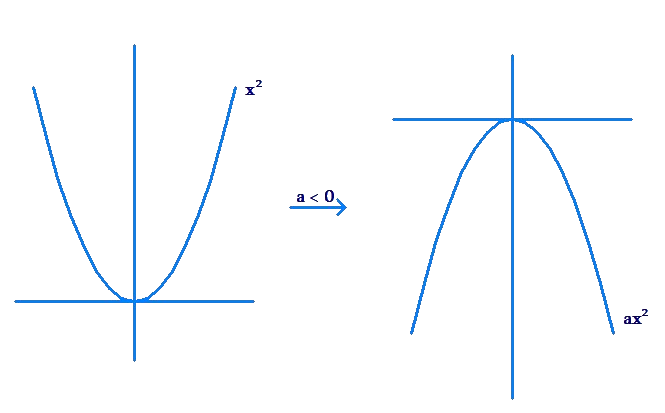All parabolas are symmetric with reference to a line called the axis of symmetry. The point where the axis of symmetry parabola intersects the curve of parabola called the vertex of the parabola.

Given three points within the plane that have different first coordinates and don't lie on a line, there's exactly one quadratic function f whose graph contains all three points.

Many quadratic functions can be graphed easily by hand using the techniques of stretching/shrinking and shifting (translation) the parabola of quadratic parent function y = x2

 Example 1

Sketch the graph of y = x2/2.

Taking up the graph of the quadratic parent function y = x2, we shrink it by a factor of 1/2. This is done by taking a point on the graph of y = x2, and drawing a new point that is one half of the way from the x-axis to that point.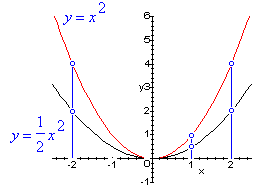Quadratic polynomial is a nothing but a polynomial having degree of 2 (note- x² in the below equation)

The standard form is f(x) =ax² + bx + c with a, b, and c being constants, or numerical coefficients, and x is an unknown variable. The first coefficient of x^2 cannot be a zero else it will become a linear function in x.

The quadratic polynomial  ax²+bx+c= 0 has two roots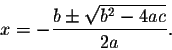The simplest parabola is y = x², whose graph is shown below. The graph is called the "Quadratic Parent Function" for parabolas, or quadratic functions.

The graph passes through the origin (0,0) and is contained in Quadrants I and II.

Various graphs of parabolas, or quadratic functions, can be derived from this graph by applying one or more transformations.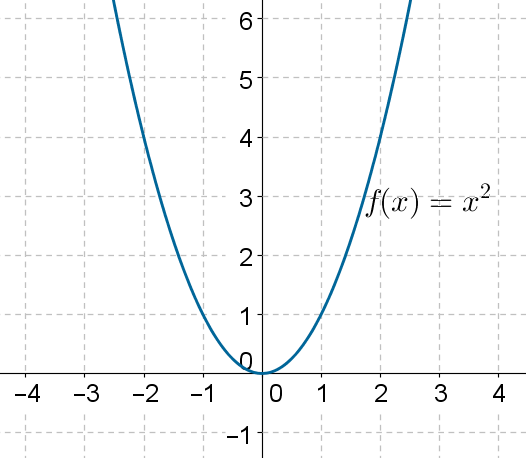## Inverse of a Quadratic Function

You know that in a direct relationship, as one variable increases, the other increases, or as one decreases, the other decreases. In an inverse relationship, instead of the two variables moving ahead  in the same direction they move in opposite directions, this means as one variable increases, the other decreases.

We have to use the following steps to seek out inverse of a quadratic function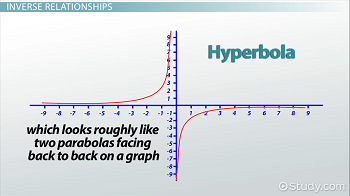### Step 1

Let f(x) be a quadratic function In the above function,f(x) to get replaced by "y" or y = f(x)

So, y = quadratic function in terms of "x"Now, the function has been defined by "y" in terms of "x"

### Step 2

Now, we've to redefine the function y = f(x) by "x" in terms of "y".Then we'll get x = g(y)

### Step 3

In x = g(y), replace "x" by f⁻¹(x) and "y" by "x".

Hence inverse of f(x) is, f⁻¹(x) = g(x)

### Finding inverse of a quadratic function

 Example

Find the inverse and its graph of the quadratic function given below.

f(x) = x

### Step 1

In the given function, allow us to replace f(x) by "y". Then, we have y = x²

### Step 2

We have to redefine y = x² by "x" in terms of "y". Then we've √y = x or x = √y

### Step 3

In x = √y, replace "x" by f⁻¹(x) and "y" by "x".Hence inverse of f(x) is f⁻¹(x) = √x

### Graphing the inverse of f(x)

We can graph the first function by plotting the vertex (0, 0). The parabola exposes, because "a" is positive.

And we get f(1) = 1 and f(2) = 4, which also are equivalent values of f(-1) and f(-2) respectively.

To graph f⁻¹(x), we've to require the coordinates of every point on the first graph and switch the "x" and "y" coordinates.

For example, (2, 4) becomes (4, 2).

We have to try to do this because the input value becomes the output value within the inverse and the other way around.

The graph of the inverse may be a reflection of the first function of the road y = x.

Graph of f(x) and its inverse f⁻¹(x)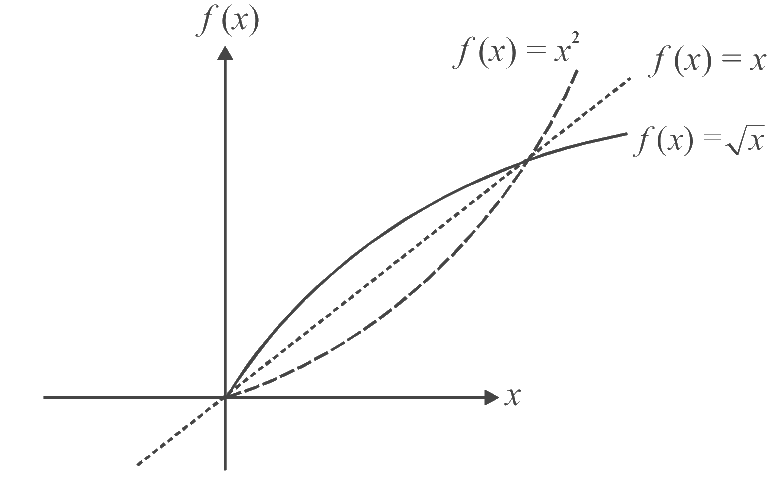Example

Find the inverse of a quadratic function given below.

f(x) = 2(x + 3)² - 4

### Step 1

In the given function, allow us to replace f(x) by "y". `Then, we have

y = 2(x + 3)² - 4

### Step 2

We have to redefine y = x² by "x" in terms of "y". Then we've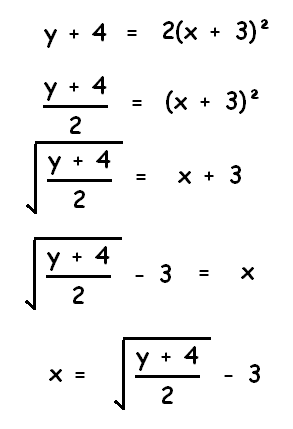### Step 3

Replacing "x" by f⁻¹(x) and "y" by "x" within the last step, we get inverse of f(x)

Hence inverse of f(x) is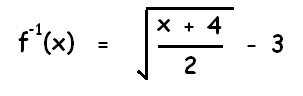Graphing the inverse of f(x)

We can graph the first function by taking (-3, -4). The parabola exposes, because "a" is positive.

And we get f(-2) = -2 and f(-1) = 4, which also are equivalent values of f(-4) and f(-5) respectively.

To graph f⁻¹(x), we've to require the coordinates of every point on the first graph and switch the "x" and "y" coordinates.

For example, (-1, 4) becomes (4, -1).

We have to try to do this because the input value becomes the output value within the inverse and the other way around.

## What are the Properties of Quadratic Function?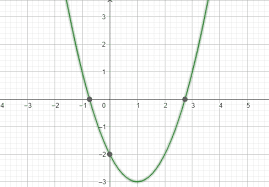• The domain of a quadratic function is all real numbers.

• Every quadratic function f(x) = ax2+ bx + c are often written as f(x) = a(x − h)2+ k, where h = − b2a and k = 4ac−b24a . So every quadratic function is simply just like the function f(x) = x 2, but transformed.

• For the quadratic function f(x) = ax2+bx+c, the vertex is usually − b2a , f − b2a and therefore the axis of symmetry is x = − b2a .

If a > 0 then the graph exposes and therefore the vertex is that the lowest point (minimum).

If a < 0 then the graph opens down and therefore the vertex is that the highest point (maximum).

• The amount of x-intercepts of a quadratic function depends on whether the graph exposes or down and it also depends on whether the vertex is above or below the x-axis. 1.

If the graph of a quadratic function exposes and therefore the vertex is below the x-axis or if the graph opens down and the vertex is above the x-axis, then there'll be two x-intercepts.

### Discriminant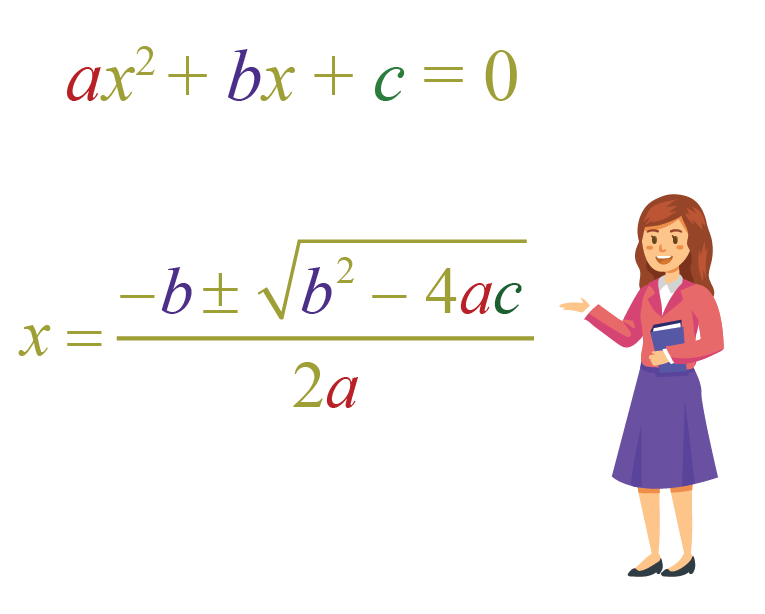The discriminant of any equation in any degree plays a crucial role in determining the roots of that equation. Just in case of a quadratic with a positive discriminant, the roots are real while a 0 discriminate indicates one real root. A negative discriminant indicates imaginary (complex number format) roots.

The quantity Δ = b2 - 4 a c under the novel above is named the discriminant and provides important information about the amount and nature of the solutions to the quadratic to be solved.

Three cases are possible:

If Δ > 0, the equation has 2 real solutions.
If Δ = 0, the equation has 1 real solution.
If Δ < 0, the equation has 2 conjugate complex solutions.

### Question 1

How to find discriminant for the quadratic given below?

x 2 + 3 x = 4

### Solution

Rewrite the given equation with its right term adequate to zero.

x 2 + 3 x - 4 = 0

Find the discriminant Δ= b2 - 4 a c

Δ= b2 - 4 a c = 32 - 4(1)(-4) = 25

Since the discriminant is positive, the equation has two real solutions given by.

x1 = ( -3 + √25 ) / (2*1) = ( -3 + 5 ) / 2 = 1

x2 = ( -3 - √25) / (2*1) = (-3 - 5) / 2 = - 4

## Summary

This lesson deals with equations involving quadratic polynomials, i.e. polynomials of degree two. Quadratic equations are equations of the form y = ax2 + bx + c or y = a(x - h)2 + k.

The shape of the graph of a quadratic equation is a parabola. The first section of this chapter explains how to graph any quadratic equation of the form y = a(x - h)2 + k, and it shows how varying the constants a, h, and k stretches and shifts the graph of the parabola We also discussed.

To master this topic like any other in mathematics understanding the concept, learning, and practicing is of utmost importance. Make sure you put in a regular 45 minutes of practice everyday to master any topic. You can get better at math with us at Cuemath, sign up for a free trial.

## What is a quadratic function?

Quadratic functions are relations of the form y = ax2 + bx + c or y = a(x - h)2 + k.

## Is a parabola a function?

Yes, parabola is a quadratic function. It has the form y = ax2 + bx + c or y = a(x - h)2 + k.